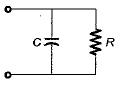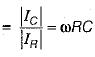Courses

# Frequency Response & Resonance - 1

## 10 Questions MCQ Test Topicwise Question Bank for Electrical Engineering | Frequency Response & Resonance - 1

Description
This mock test of Frequency Response & Resonance - 1 for Electrical Engineering (EE) helps you for every Electrical Engineering (EE) entrance exam. This contains 10 Multiple Choice Questions for Electrical Engineering (EE) Frequency Response & Resonance - 1 (mcq) to study with solutions a complete question bank. The solved questions answers in this Frequency Response & Resonance - 1 quiz give you a good mix of easy questions and tough questions. Electrical Engineering (EE) students definitely take this Frequency Response & Resonance - 1 exercise for a better result in the exam. You can find other Frequency Response & Resonance - 1 extra questions, long questions & short questions for Electrical Engineering (EE) on EduRev as well by searching above.
QUESTION: 1

### In a double tuned circuit, consisting of two magnetically coupled, identical high Q tuned circuits, at the resonance frequency of either circuit, the amplitude response has

Solution:

Since quaiity factor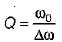and Q is high therefore, the resonant frequency ω0 will be high. Hence, the amplitude response will have a peak always

QUESTION: 2

### A network function contains only poles whose real-parts are zero or negative. The network is

Solution:

Poles are at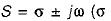can be zero or negative). The system will be stable if the poles at jω-axis are simple.

QUESTION: 3

### The minimum amount of hardware required to make a high pass filter is

Solution: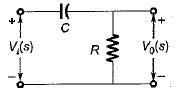Figure above shows a high-pass filter having a transfer function,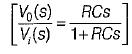QUESTION: 4

The Q-factor of a series RLC circuit having R = 10 Ω , L = 0.01 H and C = 100 μF is

Solution: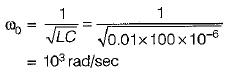Also, bandwidth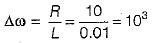∴ Quantity factor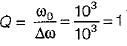QUESTION: 5

The input frequency of the given circuit for unity power factor operation is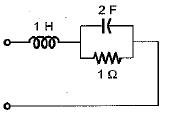Solution:

Input impedance,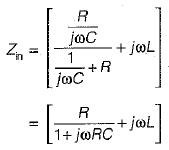or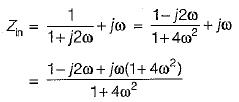For unity p.f. operation, Img(Zin) = 0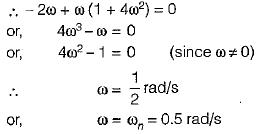QUESTION: 6

When XL is equal to Xc , then

Solution:
QUESTION: 7

If the quality factor of a series resonant circuit is increased then, its selectivity will

Solution:

Q∝  selectivity

QUESTION: 8

The steady state transfer function H(Jω) of the network shown below is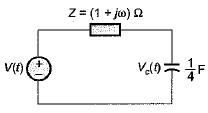Solution:

Transfer function,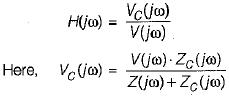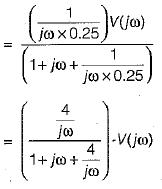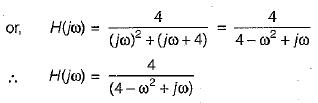QUESTION: 9

The transfer function of the circuit shown below is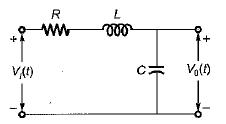Solution: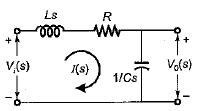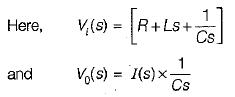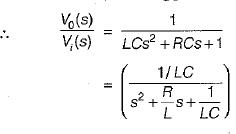QUESTION: 10

The figure of merit for a capacitor in parallel with a resistance R is given by

Solution:

Figure of merit = Q-factor